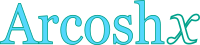## Inverse hyperbolic cosine calculator

This tool evaluates the inverse hyperbolic cosine of a number: arcosh(x). Enter the argument x below.

 x = Result: arcosh(x) =## Definitions

### General

The inverse hyperbolic cosine function, in modern notation written as arcosh(x) or arccosh(x) or cosh-1x, gives the value t (hyperbolic angle), so that:

The inverse hyperbolic cosine function accepts values not smaller than 1, because . Since the hyperbolic cosine is defined through the natural exponential function , its inverse can be defined through the natural logarithm function, using the following formula, for real x, with x≥1:

### Properties

The derivative of the inverse hyperbolic cosine function is:

The integral of the inverse hyperbolic cosine function is given by: